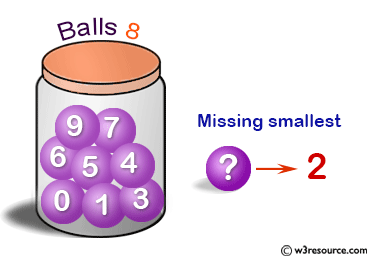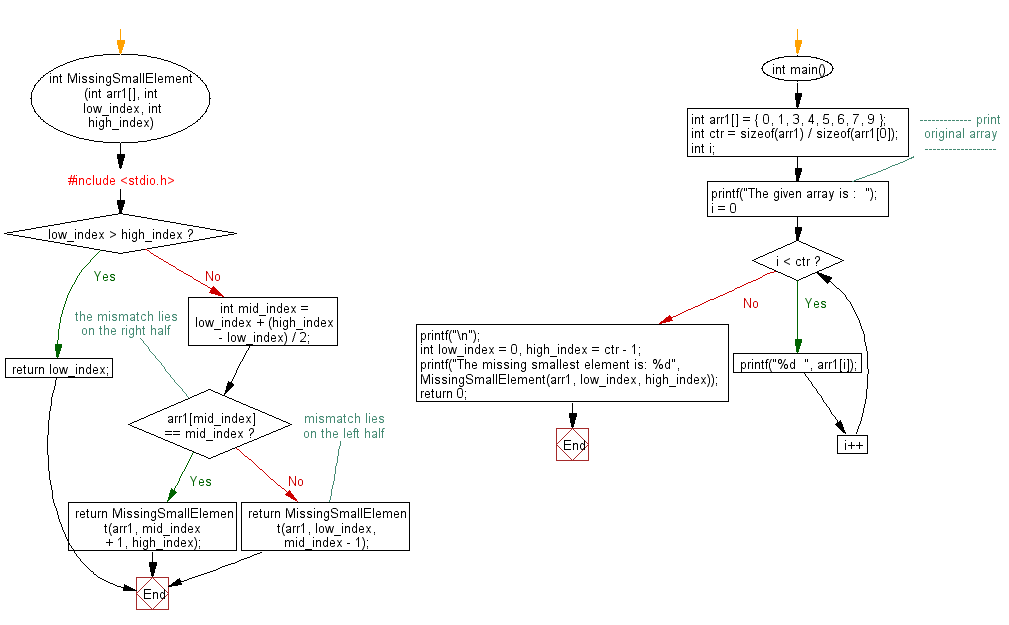﻿ C exercises: Find the smallest missing element from a sorted array - w3resource# C Exercises: Find the smallest missing element from a sorted array

## C Array: Exercise-42 with Solution

Write a program in C to find the smallest missing element from a sorted array.

Pictorial Presentation:Sample Solution:

C Code:

``````#include <stdio.h>

int MissingSmallElement(int arr1[], int low_index, int high_index)
{
if (low_index > high_index)
return low_index;
int mid_index = low_index + (high_index - low_index) / 2;

if (arr1[mid_index] == mid_index)			// the mismatch lies on the right half
return MissingSmallElement(arr1, mid_index + 1, high_index);
else										 // mismatch lies on the left half
return MissingSmallElement(arr1, low_index, mid_index - 1);
}

int main()
{
int arr1[] = { 0, 1, 3, 4, 5, 6, 7, 9 };
int ctr = sizeof(arr1) / sizeof(arr1);
int i;
//------------- print original array ------------------
printf("The given array is :  ");
for(i = 0; i < ctr; i++)
{
printf("%d  ", arr1[i]);
}
printf("\n");
//-----------------------------------------
int low_index = 0, high_index = ctr - 1;
printf("The missing smallest element is: %d",
MissingSmallElement(arr1, low_index, high_index));
return 0;
}
```
```

Sample Output:

```The given array is :  0  1  3  4  5  6  7  9
The missing smallest element is: 2
```

Flowchart:C Programming Code Editor:

Improve this sample solution and post your code through Disqus.

What is the difficulty level of this exercise?

﻿